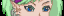-=+=- -=+=- -=+=- -=+=- -=+=- -=+=- -=+=- -=+=- -=+=- -=+=- -=+=- -=+=- -=+=- -=+=- -=+=- -=+=- -=+=- -=+=- -=+=- -=+=- -=+=- -=+=- -=+=- -=+=- -=+=- -=+=- -=+=- -=+=- -=+=- -=+=- (c) WidthPadding Industries 1987 0|327|0 -=+=- -=+=- -=+=- -=+=- -=+=- -=+=- -=+=- -=+=- -=+=- -=+=- -=+=- -=+=- -=+=- -=+=- -=+=- -=+=- -=+=- -=+=- -=+=- -=+=- -=+=- -=+=- -=+=- -=+=- -=+=- -=+=- -=+=- -=+=- -=+=- -=+=-
Socoder -> Site & Server -> Scheduled Maintenance - Oct 24th

Posted : Wednesday, 23 October 2019, 17:29
Jayenkai*phew* Sorry about the outage, there. ’’Scheduled Server Maintenance’’ that I completely forgot was happening!

### NOTICE

The site will be (/has already been) down between midnight and half past midnight on October 24th!!!

-=-=-Posted : Wednesday, 23 October 2019, 17:29
steve_ancellAnd there I was, thinking it was a server fart.
Posted : Thursday, 24 October 2019, 02:45
TrickyDid I miss something?Posted : Thursday, 24 October 2019, 04:45
JayenkaiThe server went down for about half an hour or so.
Nothing magical happened inbetweenLinode were doing some security updates to the server.

-=-=-